Journal DetailsFormat
Journal
First Published
01 Jan 2016
Publication timeframe
2 times per year
Languages
English
Copyright
© 2020 Sciendo

# Can aphids be controlled by fungus? A mathematical model

###### Accepted: 26 Apr 2019
Journal DetailsFormat
Journal
First Published
01 Jan 2016
Publication timeframe
2 times per year
Languages
English
Copyright
© 2020 Sciendo

The control of insect pests in agriculture is essential for food security. Chemical controls typically damage the environment and harm beneficial insects such as pollinators, so it is advantageous to identify targetted biological controls. Since predators are often generalists, pathogens or parasitoids are more likely to serve the purpose. Here, we model a fungal pathogen of aphids as a potential means to control of these important pests in cereal crops. Typical plant herbivore pathogen models are set up on two trophic levels, with dynamic variables the plant biomass and the uninfected and infected herbivore populations. Our model is unusual in that (i) it has to be set up on three trophic levels to take account of fungal spores in the environment, but (ii) the aphid feeding mechanism leads to the plant biomass equation becoming uncoupled from the system. The dynamical variables are therefore the uninfected and infected aphid population and the environmental fungal concentration. We carry out an analysis of the dynamics of the system. Assuming that the aphid population can survive in the absence of disease, the fungus can only persist (and control is only possible) if (i) the host grows sufficiently strongly in the absence of infection, and (ii) the pathogen transmission parameters are sufficiently large. If it does persist the fungus does not drive the aphid population to extinction, but controls it below its disease-free steady state value, either at a new coexistence steady state or through oscillations. Whether this control is sufficient for agricultural purposes will depend on the detailed parameter values for the system.

#### MSC 2010

Introduction

Aphids represent a very relevant pest in agriculture because they weaken and kill plants. To this end, they attach themselves to the most tender parts, where they are able to perforate the plant surface and then feed on the plant sap. In this way the plant receives less nutrient and suffers consequently. Aphids may be treated with pesticides, but this has negative ecological consequences; moreover, in recent times, aphids have undergone evolutionary changes in order to adapt to and to resist these human antagonistic practices, . The need of finding alternative means for better fighting them arises naturally. Biological control could be a viable solution, . It can be performed via several approaches.

For instance, specialised parasitoids, such as certain wasp species, can be used . The latter lay their eggs inside the aphid body through their skin. After hatching, the wasp larva feeds on the aphid and finally emerges to the adult stage, by which action the aphid is killed. The latter however are subject to the counteraction of common facultative bacterial symbionts, that confer resistance to these natural enemies, reducing the rate at which wasp eggs hatch, [6, 9]. The way through which the bacterial infection occurs within the aphid is not yet fully understood, altough it has been documented to occur sporadically, . Such a situation has been modeled in , focussing on aphids that “may harbour the facultative bacterial endosymbionts”, with hosts that become infected through vectors and may also get rid of their parasites. A mathematical model proposing a different transmission mechanism for the bacterial infection, through the wasp’s oviposition, has instead been studied in .

Other aphids antagonists are generally represented by predators and pathogens. This gives alternative biological ways of controlling the aphids populations. In this setting, pathogenic fungi can infect the insects with which their spores come in contact and potentially represent another possible another different way of keeping these pests in check.

In this paper, we propose a mathematical model to investigate the three-way interactions between the crops, that is the human resource, which is however hidden in the model, not explicitly taken into account as a dependent variable, aphids and fungi.

Biological setting

Aphids, along with whiteflies and scale insects, are a specialised group of herbivorous insects. Their mouth parts form a stylet, which they use to pierce plant tissues and thus gain direct access to the plant phloem and its constant stream of nutrients. This feeding habit links the insect to the plant as if the insects were just another plant organ such as a leaf. In effect, the insect population acts as an extra sink, which diverts carbon and nitrogen from the plant proper. Plant nutrients flow cleanly into the insects without the risk of accidental ingestion of pathogens. However, fungal entomopathogens have evolved to invade these sap-sucking insects not through the guts but through penetration of the insect cuticle. Infected insects upon dying turn into fungal spore-producing cadavers. Spores are actively projected from the cadaver and can infect nearby insects. A race between insect host and pathogen ensues, governed by the respective reproductive rates of the insect and the pathogen, as well as by the pathogen’s ability to spread and infect. Some spores are specialised for diapause and form resting spores, which can remain dormant in the environment for several years . In this study, we focus on aphids attacking cereals in temperate regions of the world. In such conditions, aphids have a complex life cycle shifting between winter and summer plant hosts. In the spring, aphids leave their winter host and settle in the cereal fields. Depending on weather conditions, e.g. temperature, rainfall, sunlight and the presence of aphid antagonists, such as predators, parasitoids and pathogens, aphid populations may increase extremely fast and reach crop-damaging densities. In the field, aphids reproduce parthenogenetically, giving rise to unwinged offsprings that stay in the field. As the crop ripens and the phloem dries out, an increasing proportion of the offsprings will develop wings by which they are able to leave the field. High densities of aphids as well will also induce wing formation enabling the aphids to escape intraspecific competition, . We consider the tri-trophic system consisting of cereal-aphids-fungus in the time period from spring until harvest. In natural conditions, while the fungus is always present, aphids instead immigrate from the surroundings areas in the cereal field. Aphids are lost from the system, because they are killed by natural enemies or by infection by the fungus, or by winged emigration.

Model

Let P be the biomass of plant material; S be the number of susceptible aphids; E the number of exposed aphids, i.e. infected by developing fungus but unable to transmit it, and F a measure of the amount of fungus in the environment, which is renewed whenever an infected aphid dies and decomposes. The total aphid population is given by N = S + E. Despite the last considerations of the previous section, in the model formulation we assume that the immigration and emigration effects cancel out on average, so thy can be neglected. We further lump all aphids natural enemies, other than the fungus. In this way, they contribute to form one single joint mortality factor.

The model is summarised by the compartmental diagram in Figure 1.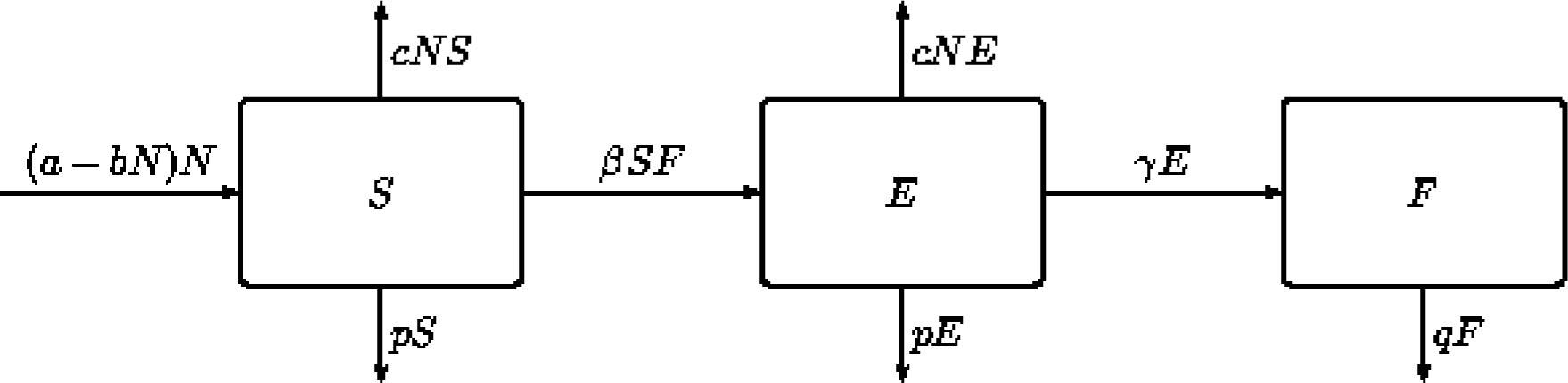Compartment diagram of the three-way (plant)-aphid-entomopathogen interactions.

The plant biomass P is assumed to grow at a rate abN, a baseline rate reduced linearly by the aphid burden, so that the equation for P is = abN. All aphid offspring, whether from a susceptible or an exposed parent, are assumed to be susceptible. They are assumed to be produced (by either type of parent) at a rate proportional to the rate at which nutrient flows in the plant phloem, and henceto the growth rate of the plants. This represents the main new feature of this model, with respect to other mainstream population models in which the populations growth rates depend only on the size of the food source. In this case instead, it becomes a function of the changes in the food structur. We scale out the constant of proportionality to arrive at the same per capita rate abN. Aphids are assumed to die from natural causes at a per capita rate p, and additionally from overcrowding at a per capita rate cN. Note that a model with different per capita death rates for S and E could be rescaled to give the model described in the following, so there is no loss of generality in taking these rates to be equal. The fungus is produced within exposed aphids, and released into the environment when they die from their infection, at a constant per capita rate γ. It degrades in the environment at a constant per capita rate q. We are interested in the dynamics of S, E and F, which are not affected by P but only by = abN, so we do not need to include the equation for P. The equations for S, E and F are as follows.

$dSdt=(a−bN)N−pS−cNS−βSF, dEdt=βSF−γE−pE−cNE, dFdt=γE−qF.$$$\begin{array}{} \displaystyle \frac{dS}{dt} = (a - b N)N - p S - c N S - \beta S F, \\\displaystyle\quad~ \frac{dE}{dt} = \beta S F - \gamma E - p E - c N E, \\\displaystyle\qquad\qquad~ \frac{dF}{dt} = \gamma E - q F. \end{array}$$

For most purposes it is easier to work with the equivalent (N, E, F) system,

$dNdt=(a−bN)N−pN−cN2−γE,dEdt=β(N−E)F−γE−pE−cNE, dFdt=γE−qF.$$$\begin{array}{} \displaystyle \,\frac{dN}{dt} = (a - b N)N - p N - c N^2 - \gamma E, \\\displaystyle \frac{dE}{dt} = \beta (N - E) F - \gamma E - p E - c N E, \\\displaystyle\qquad\qquad~\, \frac{dF}{dt} = \gamma E - q F. \end{array}$$

A positively invariant set

In this section we want to ensure that the system (1) does not have unbounded solutions, for (realistic) initial conditions in a suitable set, because otherwise the trajectories would not have biological significance.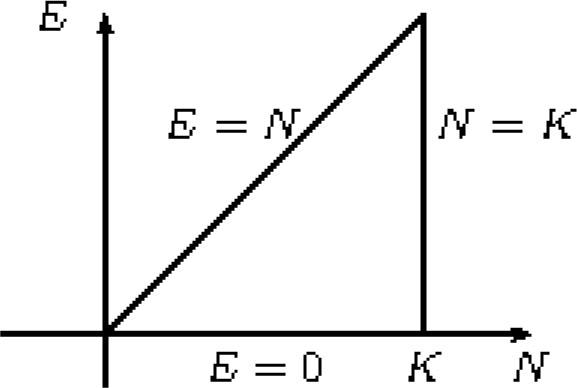A two-dimensional cross section of the positively invariant set.

We seek a positively invariant set D of the form

$D=(N,E,F)|0$$\begin{array}{} \displaystyle D = \left\{ (N,E,F) \;|\; 0 \lt N \lt K, \; 0 \lt E \lt N, \; 0 \lt F \lt \gamma K/q \right\} \end{array}$$

for some constant K. A two-dimensional cross-section of D is sketched before. We look at the various boundaries of D to check that solutions do not leave D. On the part of the boundary where N = K,

$dNdt=(a−bN)N−pN−cN2−γE=(a−p)−(b+c)KK−γE<0ifK>a−pb+c.$$$\begin{array}{} \displaystyle \frac{dN}{dt} = (a - bN)N - pN - cN^2 - \gamma E = \left( (a - p) - (b + c)K \right)K - \gamma E \lt 0 \;\; \mbox{if} \; K \gt \frac{a-p}{b+c}. \end{array}$$

On the part of the boundary where E = 0,

$dEdt=β(N−E)F=βNF≥0.$$$\begin{array}{} \displaystyle \frac{dE}{dt} = \beta (N - E) F = \beta N F \geq 0. \end{array}$$

On the part of the boundary where E = N,

$dNdt−dEdt=(a−bN)N≥0$$$\begin{array}{} \displaystyle \frac{dN}{dt} - \frac{dE}{dt} = (a - bN)N \geq 0 \;\; \end{array}$$

which holds if and only if

$N$$\begin{array}{} \displaystyle N \lt \frac ab. \end{array}$$

So for positive invariance we need K < a/b. On the part of the boundary where F = 0,

$dFdt=γE≥0.$$$\begin{array}{} \displaystyle \frac{dF}{dt} = \gamma E \geq 0. \end{array}$$

Finally, on the part of the boundary where F = γ K/q,

$dFdt=γ(E−K)≤0.$$$\begin{array}{} \displaystyle \frac{dF}{dt} = \gamma (E - K) \leq 0. \end{array}$$

So we have a positively invariant set as long as we choose K such that

$a−pb+c$$\begin{array}{} \displaystyle \frac{a-p}{b+c} \lt K \lt \frac ab, \end{array}$$

which we can do.

Steady states: existence

We shall start by analysing the steady states of the system, the solutions of = Ė = = 0. There is always a trivial steady state (N, E, F) = (0, 0, 0). We seek a disease-free (semi-trivial) steady state (N, E, F) = (N0, 0, 0). This automatically satisfies Ė = = 0, so we only need to solve

$dNdt(N0,0,0)=(a−bN0)N0−pN0−cN02=0,$$$\begin{array}{} \displaystyle \frac{dN}{dt}(N_0,0,0) = (a - bN_0)N_0 - pN_0 - cN_0^2 = 0, \end{array}$$

giving the non-trivial solution

$N0=a−pb+c.$$$\begin{array}{} \displaystyle N_0=\frac {a-p}{b+c}. \end{array}$$

This is biologically realistic (positive) if

$a>p,$$$\begin{array}{} \displaystyle a \gt p, \end{array}$$

or in other words if the basic growth rate for aphids is greater than their basic death rate. It is easy to show that if (4) does not hold, the solution of the system of differential equations tends to (0, 0, 0), so the aphids and the fungus die out, as would be expected. We shall consider from now on the case that (4) is satisfied.

We now seek non-trivial (enzootic) steady states of the form (N, E, F) = (N*, E*, F*), with none of N*, E* and F* equal to zero. The F equation gives γ E* = qF*, which with the E equation gives

$β(N∗−E∗)(γ/q)E∗=(γ+p+cN∗)E∗.$$$\begin{array}{} \displaystyle \beta (N^* - E^*) (\gamma/q) E^* = (\gamma + p + cN^*) E^*. \end{array}$$

Since E* ≠ 0, then

$βγ(N∗−E∗)=q(γ+p+cN∗),$$$\begin{array}{} \displaystyle \beta \gamma (N^* - E^*) = q(\gamma + p + cN^*), \end{array}$$

and so

$βγE∗=βγN∗−q(γ+p+cN∗)=(βγ−qc)N∗−q(γ+p).$$$\begin{array}{} \displaystyle \beta \gamma E^* = \beta \gamma N^* - q(\gamma + p + cN^*) = (\beta \gamma - q c)N^* - q(\gamma + p). \end{array}$$

From the N equation,

$(a−bN∗)N∗−pN∗−cN∗2−γE∗=0,$$$\begin{array}{} \displaystyle (a - b N^*)N^* - p N^* - c N^*{}^2 - \gamma E^* = 0, \end{array}$$

Eliminating E* between equations (6) and (7), we obtain

$β(a−bN∗)N∗−βpN∗−βcN∗2=βγN∗−q(γ+p+cN∗)$$$\begin{array}{} \displaystyle \beta(a - bN^*)N^* - \beta pN^* - \beta cN^*{}^2 = \beta \gamma N^* - q(\gamma + p + cN^*) \end{array}$$

or

$Q(N∗)=β(b+c)N∗2+(βγ−qc−β(a−p))N∗−q(γ+p)=0,$$$\begin{array}{} \displaystyle Q(N^*) = \beta(b + c)N^*{}^2 + (\beta\gamma - q c - \beta(a - p))N^* - q(\gamma + p) = 0, \end{array}$$

a quadratic equation for N* with two real roots, one positive and one negative. The negative root clearly does not give a biologically realistic steady state, and we let N* denote the positive root from now on. In order to have a biologically meaningful steady state we observe that for N* and from (5) for S* = N*E*, the values are nonnegative, but we have to check whether (6) also gives a positive value. However, observe that

$βγE∗=(βγ−qc)N∗−q(γ+p)=β(a−p)N∗−β(b+c)N∗2=βN∗(a−p)−(b+c)N∗=β(b+c)N∗(N0−N∗),$$$\begin{array}{} \displaystyle \beta \gamma E^* = (\beta \gamma - qc)N^* - q(\gamma + p) = \beta(a - p)N^* - \beta(b + c)N^*{}^2 \\\displaystyle\qquad\qquad\qquad\qquad\qquad\qquad\qquad\qquad\qquad\qquad\quad = \beta N^* \left\lbrace (a - p) - (b + c)N^* \right\rbrace = \beta (b + c) N^*(N_0 - N^*), \end{array}$$

so E* > 0 as long as N* < N0, or equivalently (see diagram) as long as Q(N0) > 0, or, given the value of N0,

$(βγ−qc)N0−q(γ+p)>0.$$$\begin{array}{} \displaystyle (\beta\gamma - qc)N_0 - q(\gamma + p) \gt 0. \end{array}$$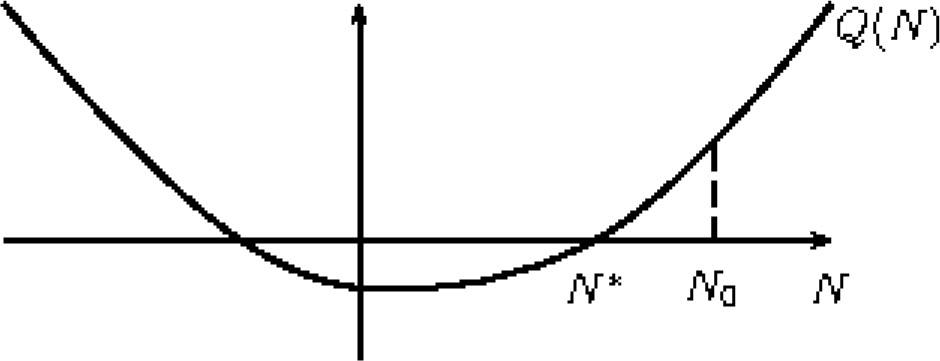A typical plot of the function Q(N) given in (8).

This is never true (for realistic N0) if βγqc < 0, so in this case there is no non-trivial steady state, and disease cannot persist in the system (at least at steady state). If β γqc > 0, it is true for N0 > and false for N0 < , where

$N^=qγ+pβγ−qc.$$$\begin{array}{} \displaystyle \hat{N} = q\frac{\gamma + p}{\beta\gamma - qc}. \end{array}$$

Equivalently, in view of (3), it is true for a > â and false for a < â, where

$a^=p+(b+c)N^=p+q(γ+p)(b+c)βγ−qc.$$$\begin{array}{} \displaystyle \hat{a} = p + (b + c)\hat{N} = p + \frac{q(\gamma + p)(b + c)}{\beta\gamma - qc}. \end{array}$$

There is a transcritical bifurcation as a increases past â, where the non-trivial steady state (N*, E*, F*) enters the positive octant by passing through the semi-trivial steady state (N0, 0, 0) when it is at (, 0, 0). Standard bifurcation theory tells us that (N*, E*, F*) becomes stable and (N0, 0, 0) unstable as a increases past â. We already know that N* < N0 for a > â. We can also show that N* increases with a, since by differentiating equation (8) we have

$dN∗da=βN∗2β(b+c)N∗+(βγ−qc)−β(a−p),$$$\begin{array}{} \displaystyle \frac{dN^*}{da} = \frac{\beta N^*}{2\beta(b+c)N^*+(\beta\gamma-qc)-\beta(a-p)}, \end{array}$$

and the formula for the solution of a quadratic shows that the denominator is positive. It follows from this that the fraction E*/N* of infected aphids decreases with a, since

$βγE∗/N∗=βγ−qc−q(γ+p)/N∗.$$$\begin{array}{} \displaystyle \beta\gamma E^*/N^* = \beta\gamma - qc - q(\gamma + p)/N^*. \end{array}$$

The bifurcation diagram with the bifurcations we have seen so far is sketched in Figure 4. There is no further bifurcation simply involving steady states, but Hopf bifurcations have not yet been ruled out.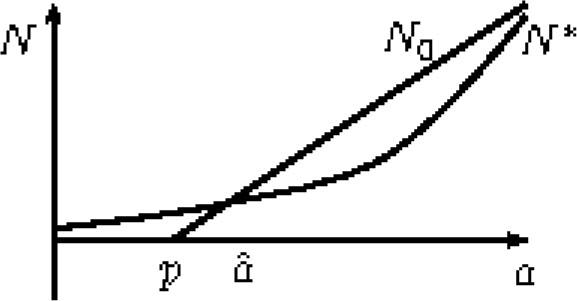Sketch of the transcritical bifurcations undergone by the system’s equilibria.

Steady states: stability

The Jacobian matrix J for the system (1) is given by

$J(N,E,F)=a−2bN−p−2cN−γ0βF−cE−βF−γ−p−cNβ(N−E)0γ−q.$$$\begin{array}{} \displaystyle J(N,E,F) = \begin{pmatrix} a - 2 b N - p - 2 c N & - \gamma & 0 \\ \beta F - c E & - \beta F - \gamma - p - c N & \beta(N - E) \\ 0 & \gamma & - q \end{pmatrix}. \end{array}$$

At (0, 0, 0), J has eigenvalues ap, −γp, and −q, so the trivial steady state is stable for a < p and unstable whenever (4) holds, as we would expect. At (N0, 0, 0), J = J0 = J(N0, 0, 0) has eigenvalues ap − 2(b + c)N0 = − (ap), unstable if a < p and stable if (4) is satisfied, and those of the two-dimensional submatrix Ĵ0 are given by

$J^0=−γ−p−cN0βN0γ−q.$$$\begin{array}{} \displaystyle \hat{J}_0 = \begin{pmatrix} - \gamma - p - cN_0 & \beta N_0 \\ \gamma & -q \end{pmatrix}. \end{array}$$

This matrix has negative trace, and so has stable eigenvalues as long as its determinant is positive, that is as long as

$q(γ+p+cN0)−βγN0=q(γ+p)−(βγ−qc)N0>0.$$$\begin{array}{} \displaystyle q(\gamma + p + cN_0) - \beta\gamma N_0 = q(\gamma + p) - (\beta\gamma - qc)N_0 \gt 0. \end{array}$$

This is always true if βγqc < 0, and is true as long as a < â if βγqc > 0. Putting everything together, (N0, 0, 0) is stable whenever a > p if βγqc < 0, and stable whenever p < a < â if βγqc > 0. We are interested in the case of βγqc > 0, which we already know is the case where the diseased steady state (N*, E*, F*) exists and is realistic. Its stability is determined by the eigenvalues of J = J* = J(N*, E*, F*), which can be simplified slightly to

$J∗=−X−γ01q(βγ−qc)E∗−1qβγN∗βS∗0γ−q,$$$\begin{array}{} \displaystyle J^* = \begin{pmatrix} - X & -\gamma & 0 \\ \dfrac 1q (\beta\gamma - qc)E^* & -\dfrac 1q \beta\gamma N^* & \beta S^* \\ 0 & \gamma & -q \end{pmatrix}, \end{array}$$

where

$X=−a+p+2(b+c)N∗$$$\begin{array}{} \displaystyle X = - a + p + 2(b + c)N^* \end{array}$$

and S* = N*E*. The eigenvalues λ of J* satisfy its characteristic equation

$λ+Xγ0−(βγ−qc)E∗/qλ+βγN∗/q−βS∗0−γλ+q=λ3+A1λ2+A2λ+A3=0,$$$\begin{array}{} \displaystyle \begin{vmatrix} \lambda + X & \gamma & 0 \\ -(\beta\gamma - qc)E^*/q & \lambda + \beta\gamma N^*/q & - \beta S^* \\ 0 & - \gamma & \lambda + q \end{vmatrix} = \lambda^3 + A_1 \lambda^2 + A_2 \lambda + A_3 = 0, \end{array}$$

where

$A1=X+βγN∗/q+q,A3=βγN∗(X+γ),$$$\begin{array}{} \displaystyle A_1 = X + \beta \gamma N^*/q + q, \qquad A_3 = \beta\gamma N^*(X + \gamma), \end{array}$$

and

$A2=1qβγN∗X+1qγ(βγ−qc)E∗+qX+βγN∗−βγS∗.$$$\begin{array}{} \displaystyle A_2 = \frac 1q \beta\gamma N^* X + \frac 1q \gamma(\beta\gamma - qc)E^* + qX + \beta\gamma N^* - \beta\gamma S^*. \end{array}$$

The (Routh--Hurwitz) conditions for stability of (N*, E*, F*) are that A1 > 0, A3 > 0, and A1A2A3 > 0. At a Hopf bifurcation, A1A2A3 = 0.

Hopf bifurcations: b and c small

We have not analysed the possibility of Hopf bifurcations in the general case, but an analysis with b and c small is possible. Let b + c = O(ε), where 0 < ε ≪ 1 . There is a bifurcation at a = p when (N0, 0, 0) enters the positive octant and becomes stable and another close to it at a = â = p + O(ε) when (N*, E*, F*) does. We now consider what happens as a increases further. We shall first show that no bifurcation occurs, and (N*, E*, F*) is stable, for a sufficiently large. Let a > p + γ, with apγ = O(1). Then, to first order, using (8), (5) and (6), we have

$N∗=a−p−γb+c=O(1/ε),βγS∗=q(γ+p+cN∗)=O(1),E∗=N∗−S∗=O(1/ε),$$$\begin{array}{} \displaystyle \quad N^* = \frac {a - p - \gamma}{b + c} = O(1/\varepsilon), \\\displaystyle\, \beta\gamma S^* = q(\gamma + p + cN^*) = O(1), \\\displaystyle\quad\, E^* = N^* - S^* = O(1/\varepsilon), \end{array}$$

and

$X=−a+p+2(a−p−γ)=a−p−2γ.$$$\begin{array}{} \displaystyle X = - a + p + 2(a - p - \gamma) = a - p - 2\gamma. \end{array}$$

so that X + γ is positive and O(1). To first order, from (15) and (16), we find

$A1=βγN∗q,A2=βγN∗X+γ+qq,A3=βγN∗(X+γ).$$$\begin{array}{} \displaystyle A_1 = \frac {\beta\gamma N^*}q, \qquad A_2 = \beta\gamma N^* \frac {X + \gamma + q}q, \qquad A_3 = \beta\gamma N^*(X + \gamma). \end{array}$$

All of these are positive and O(1/ε) since X + γ is positive and O(1). The remaining condition for stability of (N*, E*, F*) is that A1A2A3 > 0, which is satisfied since A1A2 = O(1/ε2) while A3 = O(1/ε). Hence there is no bifurcation and (N*, E*, F*) is stable whenever a > p + γ and apγ = O(1).

So (N*, E*, F*) is stable when it enters the positive quadrant at a = â = p + O(ε), and stable again when a exceeds p + γ by O(1), so it is stable for all values in between unless it loses stability through a Hopf bifurcation and regains it through a reverse Hopf bifurcation. Recall indeed that we have already shown that no other type of bifurcation can occur. We define O(1) parameters a1, b1 and c1 by b = εb1, c = εc1, and a = â + εa1 = p + (b + c) + εa1, where is given by (10). Then (8) and (6) become

$βε(b1+c1)N∗2+(βγ−εqc1−εβ(a1+(b1+c1)N^)N∗−(βγ−εqc1)N^=0, βγE∗=(βγ−εqc1)N∗−(βγ−εqc1)N^.$$$\begin{array}{} \displaystyle \beta\varepsilon(b_1 + c_1)N^*{}^2 + (\beta\gamma - \varepsilon qc_1 - \varepsilon\beta(a_1 + (b_1 + c_1)\hat{N})N^* - (\beta\gamma - \varepsilon qc_1)\hat{N} = 0, \\\displaystyle\qquad\qquad\qquad\quad~~ \beta\gamma E^* = (\beta\gamma - \varepsilon qc_1)N^* - (\beta\gamma - \varepsilon qc_1)\hat{N}. \end{array}$$

Looking for solutions $N∗=N0∗+εN1∗ and E∗=E0∗+εE1∗$$\begin{array}{} \displaystyle N^*=N_0^*+\varepsilon N_1^*~~ \text{and}~~ E^*=E_0^*+\varepsilon E_1^* \end{array}$, we obtain

$N0∗=N^,E0∗=0,$$$\begin{array}{} \displaystyle N_0^* = \hat{N}, \qquad E_0^* = 0, \end{array}$$

and

$βγN1∗=βa1N^,βγE1∗=βa1N^.$$$\begin{array}{} \displaystyle \beta\gamma N_1^* = \beta a_1 \hat{N}, \qquad \beta\gamma E_1^* = \beta a_1 \hat{N}. \end{array}$$

Definition (13) gives

$X=−a^−εa1+p+2ε(b1+c1)(N^+εN1∗).$$$\begin{array}{} \displaystyle X = - \hat{a} - \varepsilon a_1 + p + 2\varepsilon(b_1 + c_1)(\hat{N} + \varepsilon N_1^*). \end{array}$$

With X = X0 + εX1,

$X0=0,X1=−a1+2(b1+c1)N^.$$$\begin{array}{} \displaystyle X_0 = 0, \qquad X_1 = - a_1 + 2(b_1 + c_1)\hat{N}. \end{array}$$

From (15) and (16), the coefficients A1, A2 and A3 of the characteristic equation (14) for J* are given to leading order by

$A1=βγN0∗q+q,A3=εA31=εβγ2E1∗,$$$\begin{array}{} \displaystyle A_1 = \frac {\beta\gamma N_0^*}q + q, \qquad A_3 = \varepsilon A_{31} = \varepsilon\beta\gamma^2 E_1^*, \end{array}$$

and

$A2=εA21=1qεβγN0∗X1+1qεβγ2E1∗+εqX1+εβγE1∗.$$$\begin{array}{} \displaystyle A_2 = \varepsilon A_{21} = \frac 1q \varepsilon\beta\gamma N_0^* X_1 + \frac 1q \varepsilon\beta\gamma^2 E_1^* + \varepsilon q X_1 + \varepsilon\beta\gamma E_1^*. \end{array}$$

Both A1 and A3 are positive as long as $E1∗$$\begin{array}{} \displaystyle E_1^* \end{array}$ is, or equivalently as long as a > â, or a1 > 0. By Routh–Hurwitz conditions, the steady state is stable as long as A1A2A3 > 0, or equivalently as long as A1A21A31 > 0. However,

$γ(A1A21−A31)=F(N^)+G(N^)a1,$$$\begin{array}{} \displaystyle \gamma(A_1A_{21} - A_{31}) = F(\hat{N}) + G(\hat{N})a_1, \end{array}$$

where

$F(N)=1qβγN+q2(b1+c1)N,$$$\begin{array}{} \displaystyle F(N) = \left(\frac 1q \beta\gamma N + q \right)^2(b_1 + c_1)N, \end{array}$$

$G(N)=(βN−q)1qβγN+q−βγN.$$$\begin{array}{} \displaystyle G(N) = (\beta N - q)\left( \frac 1q \beta\gamma N + q \right) - \beta\gamma N. \end{array}$$

Hence A1A2A3 is positive, and so (N*, E*, F*) is stable, for a1 small and positive, as expected. It increases as a1 increases if G() > 0, and in this case (N*, E*, F*) remains stable at least in this asymptotic regime. It decreases as a1 increases if G() < 0, and in this case, it becomes zero at a1 = ã1, where

$a~1=F(N^)−G(N^).$$$\begin{array}{} \displaystyle \tilde{a}_1 = \frac{F(\hat{N})}{-G(\hat{N})}. \end{array}$$

Using the expression (10) for , the condition G() < 0 may be simplified to give

$γ2−p2−pq>0.$$$\begin{array}{} \displaystyle \gamma^2 - p^2 - pq \gt 0. \end{array}$$

Under this condition, as a1 increases past ã1, or a increases past â + εã1, (N*, E*, F*) loses stability through a Hopf bifurcation. We have shown that it regains stability later, and that this must be through a reverse Hopf bifurcation before apγ becomes O(1), but we have not analysed this reverse bifurcation.

The basic reproduction numbe R0

The basic reproduction number R0 can be thought of as the number of infected aphids produced in each generation of the infection when an infected aphid is introduced into the disease-free system, which is the system at (N0, 0, 0). Indeed, note that in this case, an infected aphid produces fungal particles in the next infection generation, which produce infected aphids in the infection generation after that; hence, we shall consider the number of infected aphids produced over two infection generations. We assume implicitly that (N0, 0, 0) exists and is realistic, in other words that (4) holds. R0 may be shown to be the largest eigenvalue of the next-generation matrix M at (N0, 0, 0), which is given after a standard calculation by

$M=a−2bN0p+2cN00000βN0r0γγ+q+cN0.$$$\begin{array}{} \displaystyle M = \begin{pmatrix} \dfrac{a - 2bN_0}{p + 2cN_0} & 0 & 0 \\ 0 & 0 & \dfrac{\beta N_0}{r} \\ 0 & \dfrac{\gamma}{\gamma + q + c N_0} \end{pmatrix}. \end{array}$$

This matrix has eigenvalues

$λ0=a−2bN0p+2cN0,λ±=±γβN0q(γ+p+cN0).$$$\begin{array}{} \displaystyle \lambda_0=\frac{a-2bN_0}{p+2cN_0}, \quad \lambda_\pm=\pm\sqrt\frac{\gamma\beta N_0}{q(\gamma+p+cN_0)}. \end{array}$$

Note that λ0 < 1, since

$(a−2bN0)−(p+2cN0)=a−p−2(b+c)N0=(a−p)−2(a−p)=−(a−p)<0.$$$\begin{array}{} \displaystyle (a - 2bN_0) - (p + 2cN_0) = a - p - 2(b + c)N_0 = (a - p) - 2(a - p) = -(a - p) \lt 0. \end{array}$$

If λ+ < 1, then all eigenvalues of M are less than 1, so R0 < 1, the disease cannot invade, and the disease-free steady state is stable. On the other hand if λ+ > 1, then R0 = λ+ > 1, and the fungus invades the disease-free steady states.

In the case of interest, $R02$$\begin{array}{} \displaystyle R_0^2 \end{array}$ may be written as

$R02=γβN0q(γ+p+cN0).$$$\begin{array}{} \displaystyle R_0^2 = \frac{\gamma \beta N_0}{q(\gamma + p + cN_0)}. \end{array}$$

This may be interpreted as follows, with arguments that can be made mathematically rigorous. An infected aphid introduced into the system at (N0, 0, 0), the primary, leaves the infected aphid (E) class at rate γ + p + cN0 and so remains in the E class for a time 1/(γ + p + cN0), on average. While it is in the class it produces fungus F at rate γ, so it produces on average γ/(γ + p + cN0) fungal particles. Each fungal particle leaves the F class at rate q, so remains in the F class for a time 1/q, on average. While it is in the class, it produces infected aphids E at rate βN0, so it produces on average βN0/q infected aphids. Hence the primary infected aphid produces on average $R02$$\begin{array}{} \displaystyle R_0^2 \end{array}$ infected aphids two generations later, so R0 per generation.

The condition for invasion, R0 > 1, may be written as

$γβN0>q(γ+p+cN0),or(βγ−qc)N0−q(γ+p)>0,$$$\begin{array}{} \displaystyle \gamma \beta N_0 \gt q(\gamma + p + c N_0), \;\; \mbox{or} \;\; (\beta \gamma - q c)N_0 - q(\gamma + p) \gt 0, \end{array}$$

or, as we saw in section 5, Q(N0) > 0, which is equivalent to N* < N0, and then to E* > 0 as well, which is identical to the condition for (N*, E*, F*) to have all components positive. Thus, whenever the diseased steady state (N*, E*, F*) is realistic, then the disease-free steady state (N0, 0, 0) is unstable to the introduction of the disease.

Numerical simulations

Using XPPAUT and Matlab, we performed some numerical simulations that are here reported. In Figure 4, the one parameter bifurcation diagram of system (1) is represented. The populations N, E and F are respectively shown as functions of the bifurcation parameter, taken as the intraspecific competition rate c. The continuous red curves respectively represent the stable equilibrium points and the black one the unstable ones. The dotted green line shows the maxima and minima values of the oscillation, once the Hopf bifurcation point is crossed. The oscillations and amplitudes are better seen in the zoomed version of Figure 6; in particular observe that while decreasing c, the amplitudes increases. Furthermore for values of c increasing past 6, the transcritical bifurcation arises for which the coexistence enzootic state turns into the disease-free state.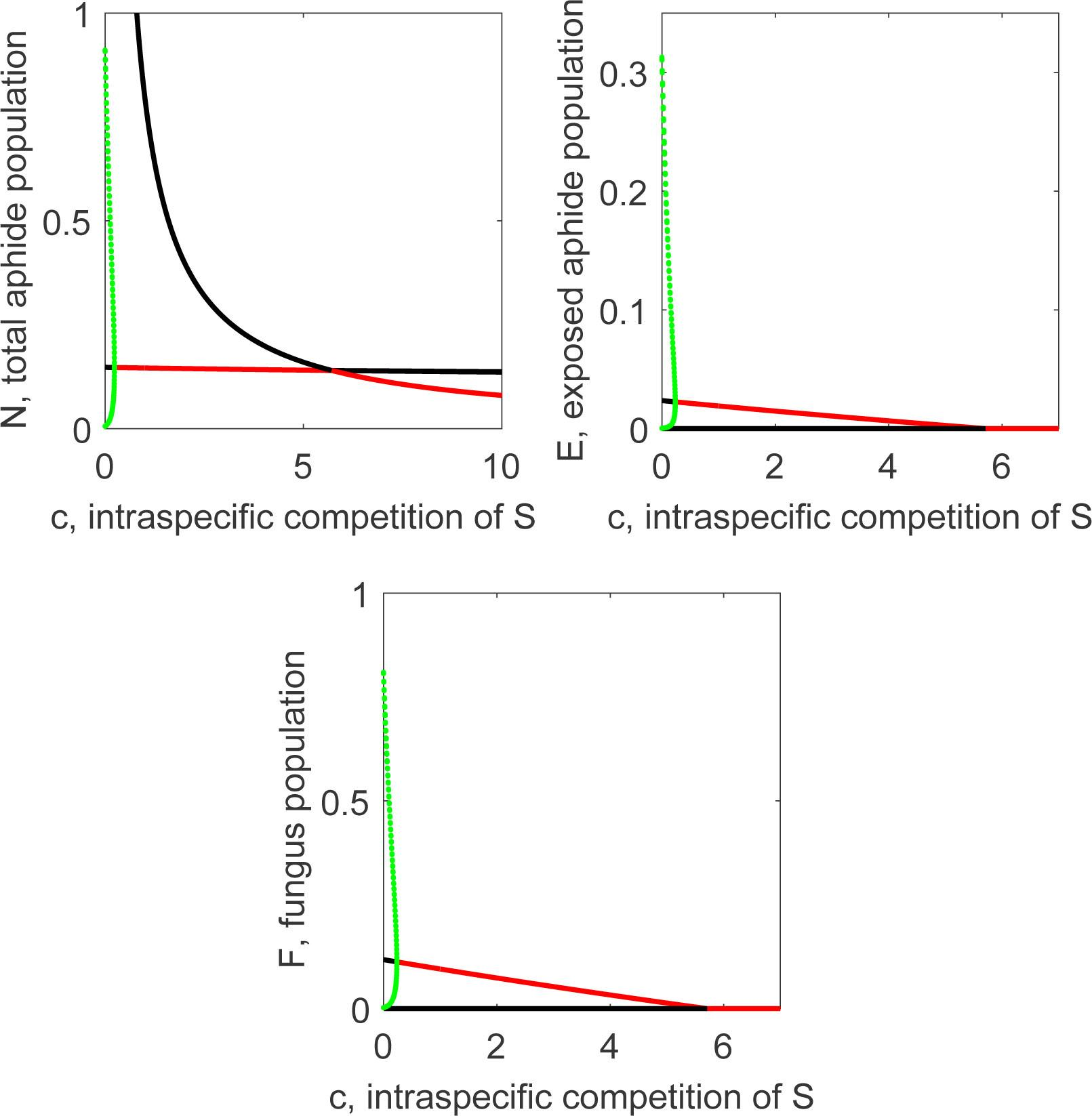One parameter bifurcation analysis of the populations N, E and F of the system (1) in terms of the parameter c, respectively. The continuous red curves represent the stable equilibrium points and the black one the unstable ones. The dotted green curve represent the maximum and minimum values of the oscillations triggered by the Hopf bifurcation. The other parameters values are β = 10, q = 1, γ = 5, b = 0.002, a = 2 and p = 1.2. The initial conditions are N = 1, E = 1 and F = 1.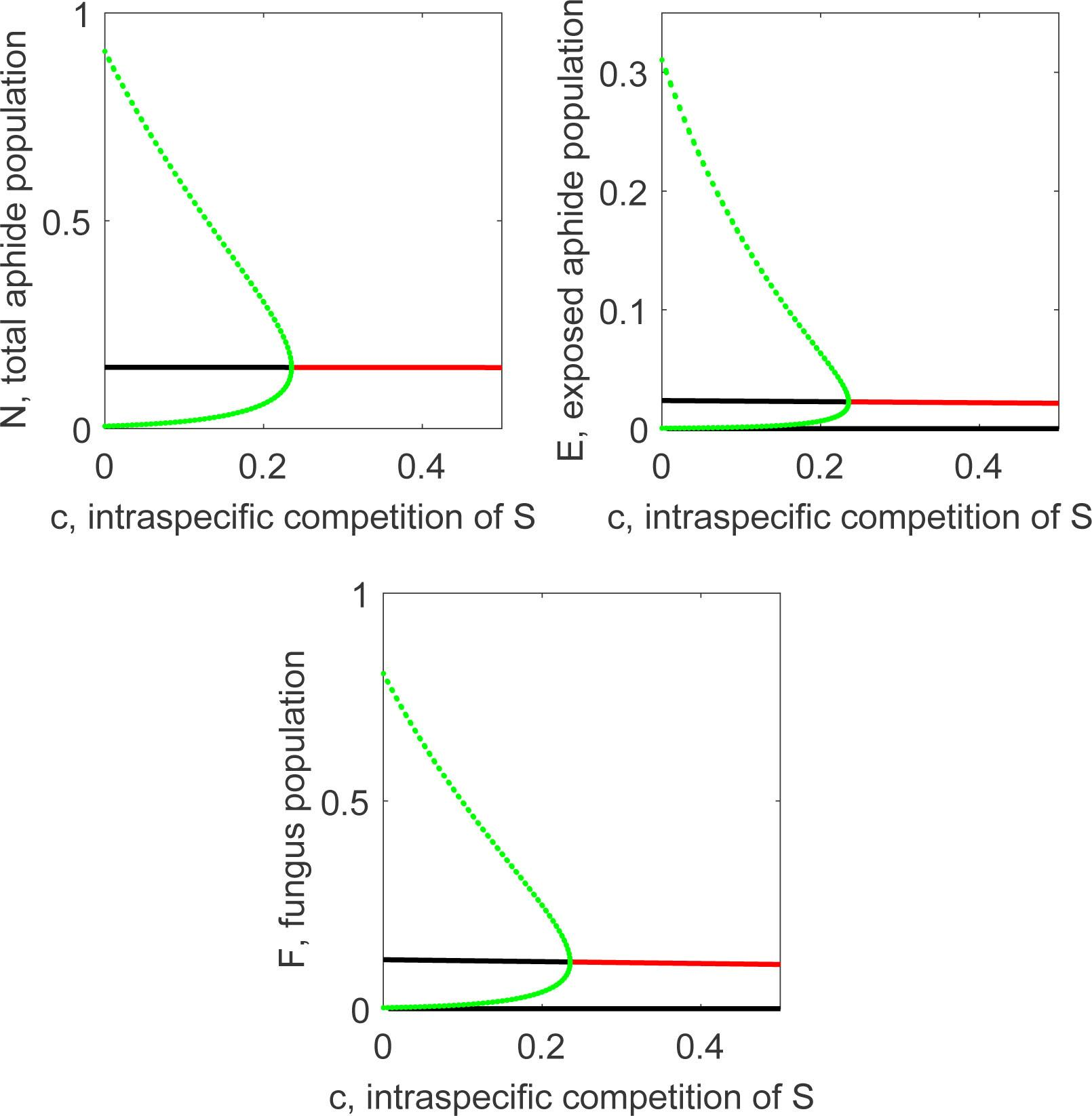Zoomed portion of the Figure 5.

In the panels of Figure 7, the possible combinations of the parameter c with the remaining six parameters of system (1) are shown. In these particular two parameter bifurcation diagrams the continuous line denotes the Hopf bifurcation points while the dashed lines represent the transcritical bifurcation points, under which the coexistence equilibrium becomes the disease-free steady state.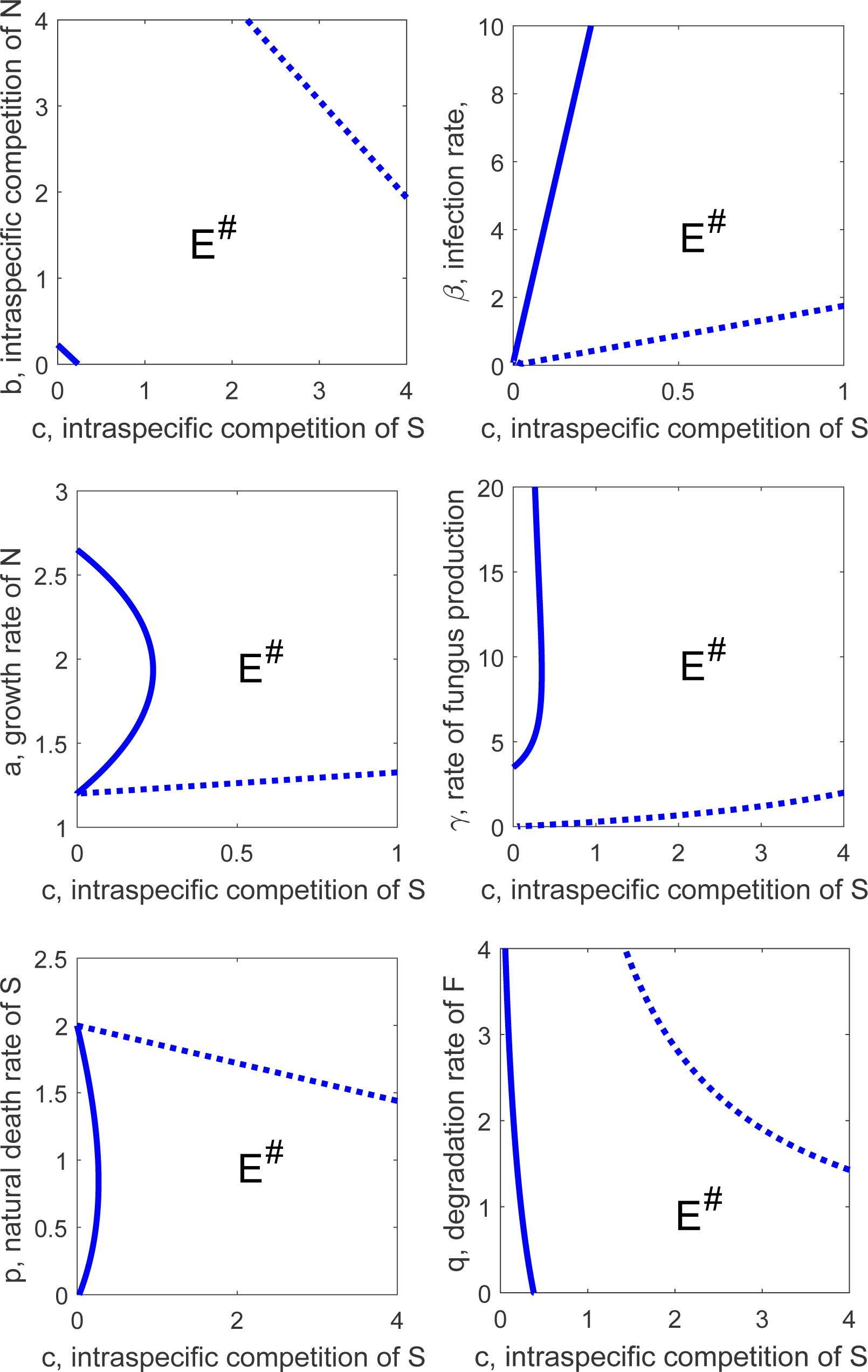Two parameter bifurcation analysis of the system (1) of the remaining six parameters as function of the bifurcation parameter c. Respectively, top to bottom and left to right, the former are b, β, s, γ, p, and q. The continuous blue line is the Hopf bifurcation curve. The dashed blue line represents the transcritical bifurcation from the coexistence equilibrium to disease-free steady state.

Conclusions

The three-way interaction ecosystem formed by crops, aphids and fungi has been shown to possess only three possible equilibria. Namely, ecosystem collapse, the disease-free state and the enzootic coexistence point. Furthermore, coexistence may occur also through persistent oscillations, demonstrated by the results of the numerical simulations.

More specifically, the system dies out unless the parameter a driving the underlying growth of the system exceeds the death rate parameter p. If a > p, the system tends to an infection-free steady state (N0, 0, 0) unless the transmission parameters are sufficiently high (βγ > qc) for infection to persist. Even if the transmission parameters are high, βγ > qc, the growth parameter a must exceed a threshold value â (with â > p) for infection to become enzootic in the system. It may be, again for βγ > qc, that the enzootic steady state (N*, E*, F*) is stable for all values of a above â. However, in some parameter regimes, particularly if b and c are small and γ, p and q satisfy a certain condition, it may be that, as a increases, this steady state loses its stability through a Hopf bifurcation and then regains it through a reverse Hopf bifurcation. Between these bifurcations we expect to see periodic solutions of the system.

The role of the parameter a in the race between host and pathogen is interesting. Although high a favours the host by increasing N*; it also favours the pathogen by increasing E*, because of course the host is an essential resource for the pathogen. However, from (11) although high, a decreases the fractionE*/N* of infected hosts. The host cannot escape the pathogen by growing quickly, but it can reduce its effect on the host population, so that biological control of a fast-growing pest will be difficult.

More generally, the biocontrol of crops in this situation should aim at obtaining the coexistence state and avoiding the disease-free point, as the infection is damaging the crop pests, and therefore it is useful to preserve and improve the harvesting. In the case of persistent oscillations on the other hand, the troughs should possibly be kept at a high level, to prevent the population of pathogenic fungi from disappearing.Compartment diagram of the three-way (plant)-aphid-entomopathogen interactions.A two-dimensional cross section of the positively invariant set.A typical plot of the function Q(N) given in (8).Sketch of the transcritical bifurcations undergone by the system’s equilibria.One parameter bifurcation analysis of the populations N, E and F of the system (1) in terms of the parameter c, respectively. The continuous red curves represent the stable equilibrium points and the black one the unstable ones. The dotted green curve represent the maximum and minimum values of the oscillations triggered by the Hopf bifurcation. The other parameters values are β = 10, q = 1, γ = 5, b = 0.002, a = 2 and p = 1.2. The initial conditions are N = 1, E = 1 and F = 1.Zoomed portion of the Figure 5.Two parameter bifurcation analysis of the system (1) of the remaining six parameters as function of the bifurcation parameter c. Respectively, top to bottom and left to right, the former are b, β, s, γ, p, and q. The continuous blue line is the Hopf bifurcation curve. The dashed blue line represents the transcritical bifurcation from the coexistence equilibrium to disease-free steady state.

Hajek, A.E.; Eilenberg, J. Natural enemies (2018), An introduction to biological control. Cambridge University Press: UK.HajekA.E.EilenbergJ.Natural enemies2018An introduction to biological control.Cambridge University PressUKSearch in Google Scholar

Dixon, A.F.G. Cereal aphids as an applied problem (1987), Agricultural Zoology Reviews, 2, 1-57.DixonA.F.G.Cereal aphids as an applied problem1987Agricultural Zoology Reviews2157Search in Google Scholar

Gehrer, L., Vorburger, C., (2012) Parasitoids as vectors of facultative bacterial endosymbionts in aphids. Biology letters, 8, 613-615.GehrerL.VorburgerC.2012Parasitoids as vectors of facultative bacterial endosymbionts in aphidsBiology letters8613615Search in Google Scholar

Kwiatkowski, M., Vorburger, C., Associate Editor: Ary, A.H. Editor: Judith, L.B., (2012) Modeling the Ecology of Symbiont-Mediated Protection against Parasites. The American Naturalist, 179, 595-605.KwiatkowskiM.VorburgerC.AryA.H.JudithL.B.2012Modeling the Ecology of Symbiont-Mediated Protection against ParasitesThe American Naturalist179595605Search in Google Scholar

Malloch, G., Pickup, J., Highet, F., Foster, S., Williamson, M., Fenton, B., (2016) Assessment of the spread of pyrethroid resistant Sitobion avenae in the UK and an update on changes in the population structure of ıMyzus persicae in Scotland. Proceedings Crop Protection in Northern Britain, 223-228.MallochG.PickupJ.HighetF.FosterS.WilliamsonM.FentonB.2016Assessment of the spread of pyrethroid resistant Sitobion avenae in the UK and an update on changes in the population structure of ıMyzus persicae in ScotlandProceedings Crop Protection in Northern Britain223228Search in Google Scholar

Oliver, K.M., Smith, A.H., Russell, J.A., (2014) Defensive symbiosis in the real world – advancing ecological studies of heritable, protective bacteria in aphids and beyond. Functional Ecology, 28, 341-355.OliverK.M.SmithA.H.RussellJ.A.2014Defensive symbiosis in the real world – advancing ecological studies of heritable, protective bacteria in aphids and beyondFunctional Ecology28341355Search in Google Scholar

Vorburger, C., (2017) Symbiont-conferred resistance to parasitoids in aphids–Challenges for biological control. Biological Control, 10.1016/j.biocontrol.2017.1002.1004.VorburgerC.2017Symbiont-conferred resistance to parasitoids in aphids–Challenges for biological controlBiological Control10.1016/j.biocontrol.2017.1002.1004

Zytynska S.E., Venturino E., (2019) Modelling the role of vector transmission of aphid bacterial endosymbionts and the protection against parasitoid wasps, to appear in: Rubem Mondaini (Editor) Biomat 2018, Springer.ZytynskaS.E.VenturinoE.2019Modelling the role of vector transmission of aphid bacterial endosymbionts and the protection against parasitoid wasps, to appear in: Rubem Mondaini (Editor) Biomat 2018SpringerSearch in Google Scholar

Zytynska, S.E., Weisser, W.W., (2016) The natural occurrence of secondary bacterial symbionts in aphids. Ecol Entomology, 41, 13-26.ZytynskaS.E.WeisserW.W.2016The natural occurrence of secondary bacterial symbionts in aphidsEcol Entomology411326Search in Google Scholar